top of page

# OTL Vacuum Tube Amplifier

For this project, I wanted to build a vacuum-tube-based amplifier for my office computer. I also wanted to use an unconventional design. I also didn't need a lot of power output - perhaps 1-2 watts.

The challenge with tube amplifiers is that tubes are high voltage, low current devices - high impedance - while standard loudspeakers are low voltage, high current devices - low impedance. Thus, typically, a tube amplifier has an output transformer to change the high impedance the tubes like for the low impedance of the speaker. I didn't have a transformer in the junkbox and I wanted to try something unusual.

The result is an "output transformer-less" (OTL) amplifier. There are many possible circuits, none of which are particularly efficient, but they can be made to work. One common circuit is the "White Cathode Follower" or "WCF" amplifier. The original patent and some discussion can be found here

I found a wealth of information in two extremely readable and thorough books written by Morgan Jones. The first is Valve Amplifiers (4th ed) and the second is Building Valve Amplifiers (2nd ed); I will refer to these as VA and BVA, respectively when I cite from them.

The WCF is based on the classic cathode follower circuit below. Cathode followers have very high input impedance, a gain of slightly less than 1.0, and a very low output impedance. It's the low output impedance that's key. A cathode follower is shown below: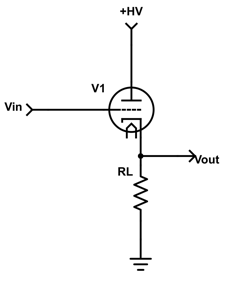The problem with a cathode follower is that, if you want some significant output power at Vout, it can be very inefficient because RL is a fixed resistor. On the positive 1/2 cycles of the input, the current flows from HV through V1 to the output and you want as high an RL as possible so all the current goes to Vout. On the negative 1/2 cycles, V1 is off and the output must sink current from the load through RL. More power output requires a lower RL. So, there's a conflict.

What you really need is an RL that has a high resistance during the positive 1/2 of the cycle and a low resistance during the negative 1/2 of the cycle. The WCF uses a second tube as this variable resistance (VA 114-118). This is shown with the DC operating point voltages below: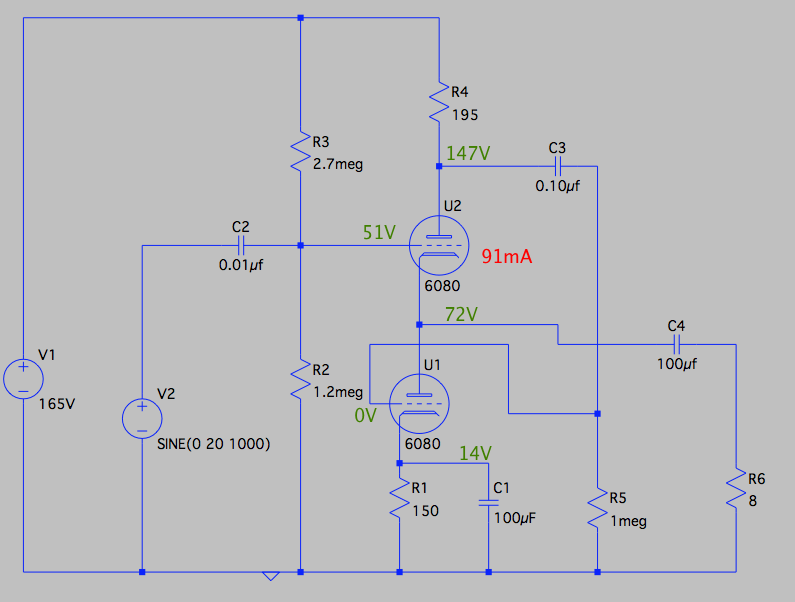In the schematic, R6 is the 8-ohm speaker load.

The input signal is V2 - a +/- 20V (40V P-P) 1kHz sine wave).

U2 is the cathode follower and U1 is the "variable RL tube". On the positive 1/2 cycle, U2 is conducting so the voltage on the plate of U2 is reduced. This is coupled to the grid of U1 through C3, causing U1 to be "off" - providing the high RL so most of the current goes out through C4 to the load. On the negative 1/2 cycle, U2 is not conducting, so the voltage on its plate rises, turning on U1 - providing a low RL so the circuit can sink significant current from the load.

The WCF is very inefficient - see later - but it can drive low impedance loads more efficiently than a standard cathode follower.

I then needed some tubes that could handle a lot of current at a low voltage. I wanted to keep the HV as low as possible, since anything higher than what's needed to get the tubes conducting is wasted in heat. I did have a number of 6080 tubes in my junkbox. These are very high current (125mA) triodes designed to be the pass tube in a voltage regulator. They can pass significant current even at low plate voltages (see below), so they're a good choice for a WCF.I wanted to model the 6080 in LTSpice and I got a model from here. I looked under 6080 and used the "modified Koren model" and edited the "^" exponentiation operator to "pow".

.subckt 6080  P G K
Cgp G P  8.6p
Ci  G K  5.5p
Co  P K  2.5p
Bp  P K  I=(1.013415356E-3)*pow(uramp(V(P,K)*ln(1.0+(-0.1045434407)+exp((2.33820774)+(2.33820774)*((3.093245565)+(0.7741425401E-3)*V(G,K))*V(G,K)/sqrt(pow(31.33315623,2)+pow(V(P,K)-(2.56937953),2))))/(2.33820774)),(1.343720741))
.ends 6080

I would then need to drive the WCF stage with significant current for the 6080s, so I settled on a standard cathode follower. I had a few 6F8 dual triodes that had a grid cap on the top. I could have used any of a wide range of triodes or even pentodes, but I wanted the cool look of a cap on the top of the tube so I used the 6F8. (If you can't get a 6F8, a 6J5 has the same specs.

I found a 6F8 model on line (I can't remember where) and edited it as above:

.subckt 6F8  P G K
Bp  P K  I=(0.02715505996E-3)*uramp(pow(V(P,K)*ln(1.0+(-0.3065895506)+exp((3.297018466)+(3.297018466)*((21.44535187)+(-105.2188672E-3)*V(G,K))*V(G,K)/sqrt(pow(33.7201302,2)+pow(V(P,K)-(7.789135657),2))))/(3.297018466),1.295896224))
.ends 6F8 curve

I also wanted to add significant negative feedback to help reduce distortion. After many rounds of design and playing in LTSpice, I came up with the design below.

The most critical values are R8, R12, and R15. R8 and R12 set the operating point for U4 and R15 sets the level of drive to U4. The three resistor values interact a lot, so it took a lot of fiddling to:

• balance the currents in U4 and U5 so they were equal and opposite

• set the operating points for U4 and U5 so they never went into clipping

• set the drive to U4 and U5 so they don't go into clipping

The currents and voltages shown from the simulation are resting +/- signal.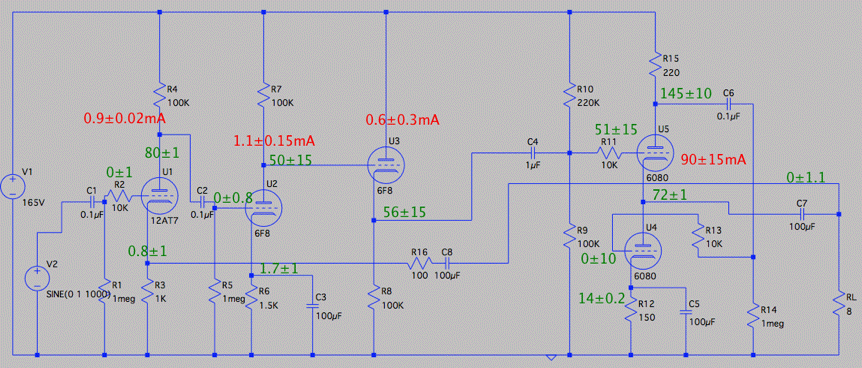It was now time to enter the real world, so I built just the output circuit - U4 and U5 -  on a breadboard. The DC operating levels were all within 5% of the simulation (!), the gain was as expected (1/15), and the response was very linear.But when I added the rest of the circuit, it oscillated like crazy! So, I removed C4 since the DC voltages on either side are the ~ the same and capacitors add phase shifts that can help make it oscillate. This meant I could dispense with R9 and R10 since they were establishing bias for U5. I also added a capacitor from U5's grid to ground to bypass the HF oscillations. I also reduced the feedback by increasing R16. This made version 7: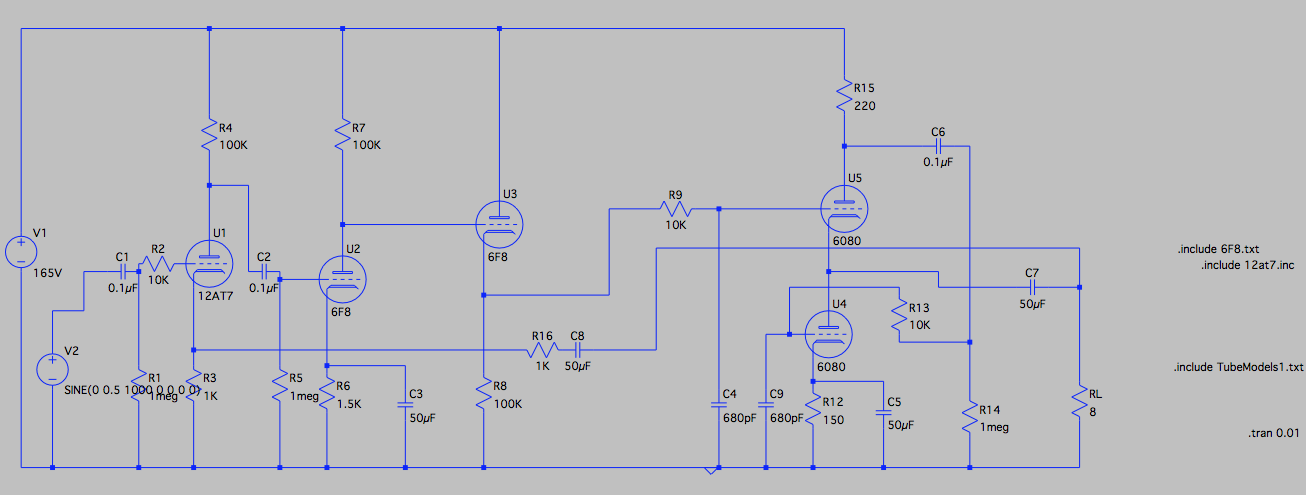The circuit works like this:

• The input level from the PC (typically 1V P-P) is coupled in through C1. R1 is the grid leakage resistor and R2 serves as a "grid stopper" to prevent VHF oscillations (VA 468).

• The first stage has an open-loop gain of about 25. The cathode bias resistor (R3) is unbypassed since the feedback is applied to the cathode of U1.

• The second stage (U2) has an open loop gain of about 15. The cathode bias resistor is bypassed to increase the AC gain.

• The third stage (U3) has an open-loop gain of slightly less than 1 and drives the output stage (U4 and U5).

• R9 and R13 are grid-stopper resistors​

• C8 and C9 are to reduce high-frequency oscillations

• C6 couples the output of U5 to the input of U4 to make the WCF.

• C7 is the output coupling capacitor to remove the DC from the output.

• Feedback is through R16 (which sets the amount of feedback) and C8 (which removes the DC).

This looked great but the power output was a little anemic. So double the 6080s - replace U4 and U5 with two tubes each in parallel. You also need to: reduce U4's grid resistor to 500K since now two tubes in parallel and reduce R12 and R15 since the current will be double.

After much simulation and breadboarding, came up with WCF10: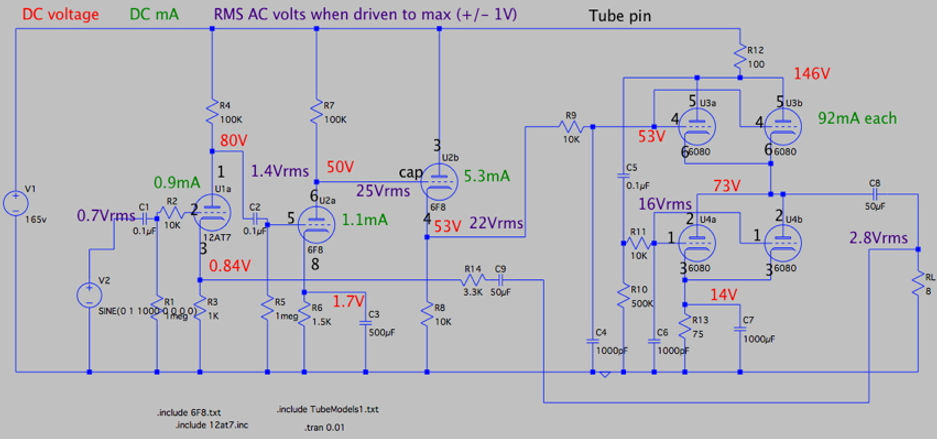I then needed an HV power supply. It must produce 165V at at least 500mA for two channels. MeanWell LPF-40-54 each produce 54V at 760mA. Three of these in series would be plenty. I used these switching power supplies since the HV supply must have a very low output impedance or the WCF circuit won't work well - effectively, the PS's output resistance is in series with R12, so if it's not much less than R12, the circuit won't work as advertised.Here's how it looked on the bench. Clockwise from left:

• HP-209A signal generator

• Tektronix TDS-210 'scope

• MeanWell HV PS under the scope

• Kepco HV power supply - only used the filament supply

• Speaker on extreme rightAfter a lot of fiddling and simulating to get good frequency response, ended up making C8 be 150uF to improve the low frequency response.

Built it into a box with the 3 MeanWell HV PS and an RL-1052 45 second delay that turns the HV on 45 seconds after the filament supply is on.

One problem: had wanted to use a 12V switching power supply to drive the filaments but it won't light the tubes! When cold, the filament currents are so high that they trigger the foldback current limiting of the supply so the tubes never get hot enough. So I went with a standard filament transformer: I need 5.75A for all the tubes, so I got one rated for 8A.

The final product looks like this:The milliamp meters are one for each channel and the center voltmeter measures the HV. The HV runs at exactly 162V (3 x 54V as expected) and the channels run a steady 150mA each.

I adjusted the trimmers (R14) for an overall gain of 4 - this gives the best distortion performance.

When connected to very high efficiency speakers (Klipsch Synergy B-20 with sensitivity of 92.5dB) it is plenty loud for my office computer speakers.

It puts out about 1/2 watt per channel and the THD (as measured with a QA400 analyzer) is quite low: <1% until you get right up to max power. You can tell when you're running it too hard when the plate current meter needles start wiggling - normally, they're completely steady.

The graph below is the %THD @1kHz using the QA400 analyzer:Here's the frequency response using the QA400:bottom of page